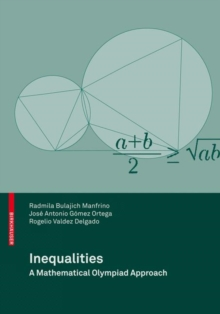Supporting your high street Find out how »
• My Account# Inequalities : A Mathematical Olympiad Approach Paperback / softback

#### Description

This book is intended for the Mathematical Olympiad students who wish to prepare for the study of inequalities, a topic now of frequent use at various levels of mathematical competitions.

In this volume we present both classic inequalities and the more useful inequalities for confronting and solving optimization problems.

An important part of this book deals with geometric inequalities and this fact makes a big difference with respect to most of the books that deal with this topic in the mathematical olympiad.

The book has been organized in four chapters which have each of them a different character.

Chapter 1 is dedicated to present basic inequalities.

Most of them are numerical inequalities generally lacking any geometric meaning.

However, where it is possible to provide a geometric interpretation, we include it as we go along.

We emphasize the importance of some of these inequalities, such as the inequality between the arithmetic mean and the geometric mean, the Cauchy-Schwarz inequality, the rearrangementinequality, the Jensen inequality, the Muirhead theorem, among others.

For all these, besides giving the proof, we present several examples that show how to use them in mathematical olympiad problems.

We also emphasize how the substitution strategy is used to deduce several inequalities.

#### Information

• Format: Paperback / softback
• Pages: 220 pages, 220 p.
• Publisher: Birkhauser Verlag AG
• Publication Date:
• Category: Algebra
• ISBN: 9783034600491

#### Other Formats

£44.99

£36.75£44.99

£37.59

£14.99

£12.74

£44.99

£38.24